# How to Generate Random Date in Excel

In some special situations we may need to generate some random dates in worksheet. We can enter date manually, but if we want to require a lot of random dates, we will spend a lot of time on manual entry, and this work is very boring. Above all, we need to find out a quick and convenient way to insert random dates in excel, this tutorial will help you to solve this problem by enter formula with RANDBEWEEN and DATE functions.

## Generate Random Date in Excel

Step 1: Select one cell, enter the formula =RANDBETWEEN(DATE(2019,1,1),DATE(2019,12,31)). RANDBETWEEN function returns data between bottom and top, DATE function returns a date. This formula will return a random date between 2019,1,1 and 2019,12,31.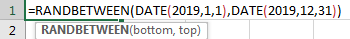Step 2: Click Enter to get the result. Verify that five digits number 43617 is displayed.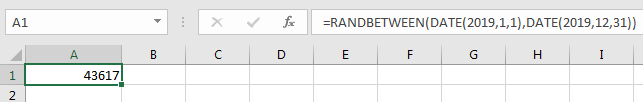Step 3: In Home ribbon, click Generate dropdown list in Number group.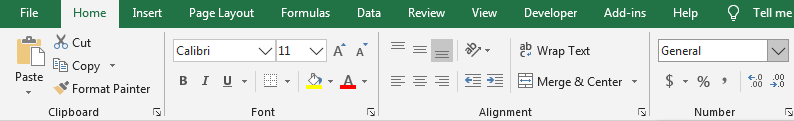Step 4: Select Short Date or Long Date format depends on your requirement.Step 5: Verify that previous number is converted to date format properly. And this date is a random date between start and end dates we entered in formula.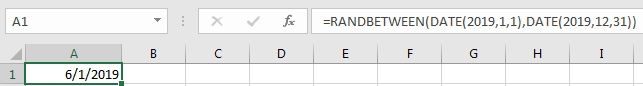Step 6: For the purpose generate multiple random dates, we just need to drag fill handle down to create more random dates. You can also copy this formula to other cells to get more random dates.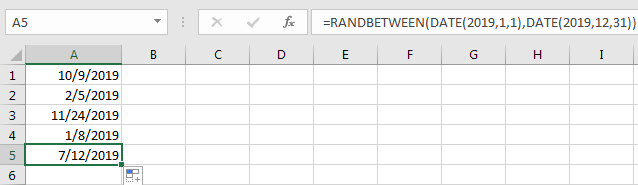Comment:

1. After step 2, you can also change number to other custom date format in Format Settings as well.

Right click to load menu, select Format Cells.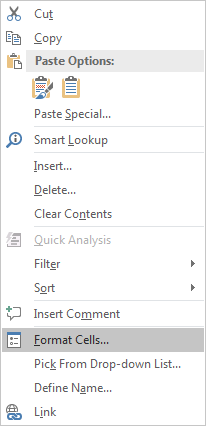Under Number tab, select Date in Category, select date format in Type, for example YYYY-MM-DD.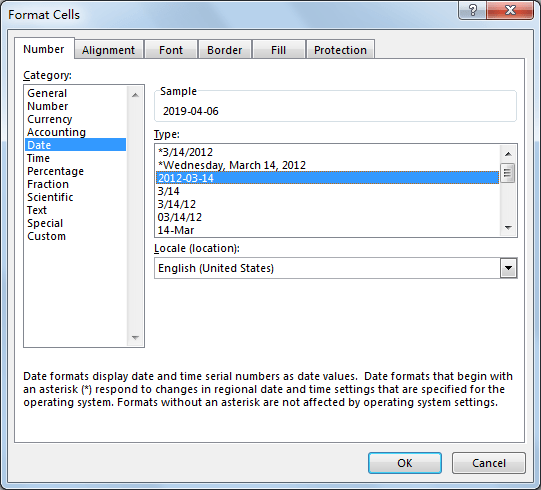After above setting, click OK. Verify that number is changed to date format properly.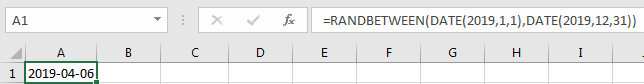2.This formula returns a date between two entered dates; it can be a weekday or weekend. If you want to only generate weekday exclude weekends, you can enter this formula =WORKDAY(RANDBETWEEN(DATE(2019,1,1),DATE(2019,12,31))-1,1). Then you can find that generated random dates are weekdays.

### Related Functions

• Excel RANDBETWEEN Function
The Excel RANDBETWEEN function returns a random integer number that is between the numbers you specify.The syntax of the RANDBETWEEN function is as below:= RANDBETWEEN (bottom,top)….
• Excel DATE function
The Excel DATE function returns the serial number for a date.The syntax of the DATE function is as below:= DATE (year, month, day)…
Related Posts

Add Days to Date in MS Excel

If you are an vavid MS Excel user, then you might have come across situations where you need to add the same or different days into the particular date, and if you have done this task manually, then let me ...

How to Sum if Date Between Two Dates in Excel

In previous article, we have introduced you the method to sum if by year, you can check “How to Sum Data if by Year in Excel” on our website for reference. But sometimes we may want to sum numbers not ...

How to Count Cells between Two Dates or Times in Excel

This post will guide you how to count cells between two dates using a formula in Excel 2013/2016. How do I count times between a given time or date range based on a condition in Excel. Is there a easy ...

How to Sum Values Based on Month and Year in Excel

We often do some summary or statistic at the end of one month or one year. In these summary tables, there are at least two columns, one column records the date, and the other column records the sales or product ...

How to Convert Text to Time in Excel

This post will guide you how to convert time string to time in Excel. Assuming that you have a list of text string in your worksheet, and you wish to convert these time string to standard time format, how to ...

Sidebar